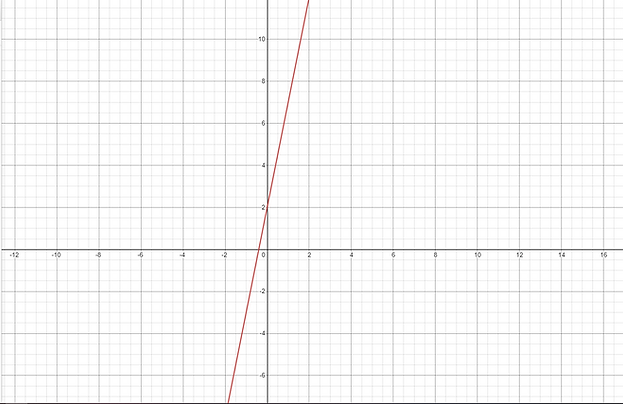top of page

# Linear Equations

In this section, we'll go over linear equations. We'll also discuss solving 1-variable equations.

## What is a Linear Equation?

A linear equation is any equation that can be put into the form y=mx+b when x and y are our unknown variables,

Example:

y = 7x - 9​

Why is this a linear equation?

As we can see, this follows the y=mx+b format, since m = 7 and b = -9 . We can graph this on the x-y plane pretty easily. For example, when (0,-9) is a point on the graph. Try it out yourself, what do you notice?

Example:

Is 2y-9x+3=4y a linear equation?

Yes! This is an example of a linear equation because we can rewrite it as 2y = -9x+3. How do we simplify further to get it into the desired form?

Example:

Graph the Equation y=5x+2

First, we see that this equation is in the form y=mx+b, which means it must be a straight line! Now, how steep is this line? Since the coefficient of the x is 5, we know that the slope will be 5. In other words, for every unit in the x direction, the value of y will increase by 5. That's why the line looks steep! Finally, let's observe the y-intercept. This is the coordinate when x=0. In this case, (0,2) is the y-intercept. With this information, we're able to fully graph the equation.

Solving Single Variable Equations:

Now that we have a grip on linear equations, let's start solving single variable equations. When solving these equations, there's one rule we always need to follow: do the same operations on both sides!

Example 1:

Solve 5x+7= -2x +19

Step 1: 5x+7+2x=-2x+19+2x

Step 2: 7x+7 = 19

Step 3: 7x+7-7 = 19-7

Step 4: 7x = 12

Step 5: x=12/7

In each step, we either added, subtracted, multiplied, or divided by the same number on both sides. This will ensure that the equation is always consistent.

You try it!

1. Solve for x in the equation 2x+6 = 19x-20

2. Solve for x in the equation 2x - 19 = 9x + 9

3. Solve for x in the equation 13x-7+30x = 9x-13 + 4(x-8)

Word Problem!

Setting up equations and solving for unknown values is an important skill, especially with word problems.

Example:

John buys some apples and pears. He buys 6 apples and 3 pears, for a total of 15 dollars. If each pear costs \$1, how much does an apple cost?

Solution:

We first need to set up an equality. Let a be the value of an apple and p the value of a pear. Then we must have

6a+3p=15 (why?). But we are given that p is 1. So we actually just have 6a+3=15. From here, we can subtract 3 from both sides to get 6a = 12. So, what's the value of a? (Hint: its 2, but show work!)

You try it!

The perimeter of a rectangle is 34 units. Its width is 6.5 units. Find the length.bottom of page